# Frank solutions for Class 9 Maths ICSE chapter 13 - Inequalities in Triangles [Latest edition]

#### Chapters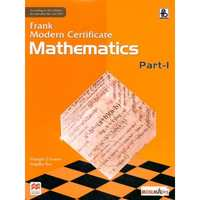## Chapter 13: Inequalities in Triangles

Exercise 13.1
Exercise 13.1

### Frank solutions for Class 9 Maths ICSE Chapter 13 Inequalities in Triangles Exercise 13.1

Exercise 13.1 | Q 1.1

Name the greatest and the smallest sides in the following triangles:
ΔABC, ∠ = 56°, ∠B = 64° and ∠C = 60°.

Exercise 13.1 | Q 1.2

Name the greatest and the smallest sides in the following triangles:
ΔDEF, ∠D = 32°, ∠E = 56° and ∠F = 92°.

Exercise 13.1 | Q 1.3

Name the greatest and the smallest sides in the following triangles:
ΔXYZ, ∠X = 76°, ∠Y = 84°.

Exercise 13.1 | Q 2.1

Arrange the sides of the following triangles in an ascending order:
ΔABC, ∠A = 45°, ∠B = 65°.

Exercise 13.1 | Q 2.2

Arrange the sides of the following triangles in an ascending order:
ΔDEF, ∠D = 38°, ∠E = 58°.

Exercise 13.1 | Q 3.1

Name the smallest angle in each of these triangles:
In ΔABC, AB = 6.2cm, BC = 5.6cm and AC = 4.2cm

Exercise 13.1 | Q 3.2

Name the smallest angle in each of these triangles:
In ΔPQR, PQ = 8.3cm, QR = 5.4cm and PR = 7.2cm

Exercise 13.1 | Q 3.3

Name the smallest angle in each of these triangles:
In ΔXYZ, XY = 6.2cm, XY = 6.8cm and YZ = 5cm

Exercise 13.1 | Q 4

In a triangle ABC, BC = AC and ∠ A = 35°. Which is the smallest side of the triangle?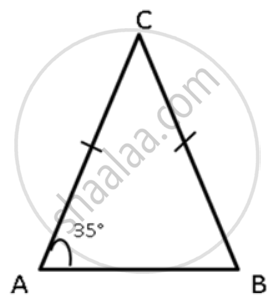Exercise 13.1 | Q 5

In ΔABC, the exterior ∠PBC > exterior ∠QCB. Prove that AB > AC.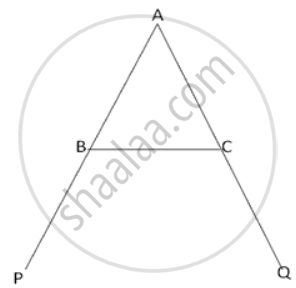Exercise 13.1 | Q 6

ΔABC is isosceles with AB = AC. If BC is extended to D, then prove that AD > AB.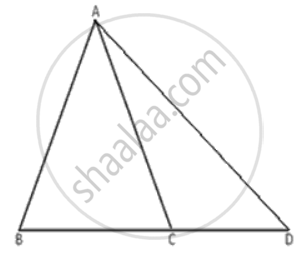Exercise 13.1 | Q 7

Prove that the perimeter of a triangle is greater than the sum of its three medians.

Exercise 13.1 | Q 8

Prove that the hypotenuse is the longest side in a right-angled triangle.

Exercise 13.1 | Q 9

D is a point on the side of the BC of ΔABC. Prove that the perimeter of ΔABC is greater than twice of AD.

Exercise 13.1 | Q 10

For any quadrilateral, prove that its perimeter is greater than the sum of its diagonals.

Exercise 13.1 | Q 11

ABCD is a quadrilateral in which the diagonals AC and BD intersect at O. Prove that AB + BC + CD + AD < 2(AC + BC).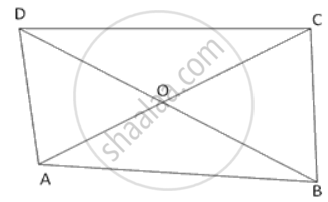Exercise 13.1 | Q 12

In ABC, P, Q and R are points on AB, BC and AC respectively. Prove that AB + BC + AC > PQ + QR  + PR.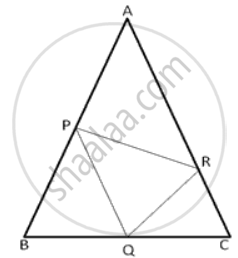Exercise 13.1 | Q 13

In ΔPQR, PR > PQ and T is a point on PR such that PT = PQ. Prove that QR > TR.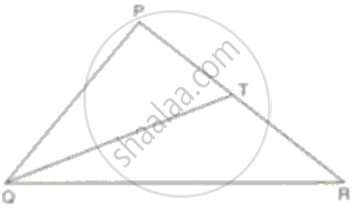Exercise 13.1 | Q 14.1

ABCD is a trapezium. Prove that: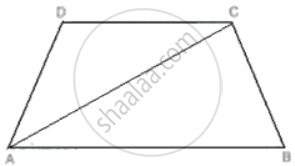CD + DA + AB + BC > 2AC.

Exercise 13.1 | Q 14.2

ABCD is a trapezium. Prove that:CD + DA + AB > BC.

Exercise 13.1 | Q 15.1

In the given figure, ∠QPR = 50° and ∠PQR = 60°. Show that : PN < RN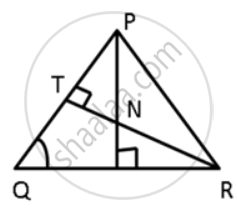Exercise 13.1 | Q 15.2

In the given figure, ∠QPR = 50° and ∠PQR = 60°. Show that: SN < SRExercise 13.1 | Q 16

In ΔABC, BC produced to D, such that, AC = CD; ∠BAD = 125° and ∠ACD = 105°. Show that BC > CD.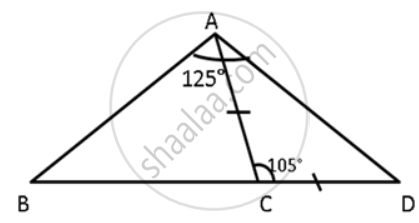Exercise 13.1 | Q 17.1

In ΔPQR, PS ⊥ QR ; prove that: PQ > QS and PQ > PS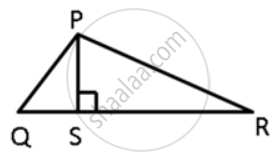Exercise 13.1 | Q 17.2

In ΔPQR, PS ⊥ QR ; prove that: PQ > QS and PR > PSExercise 13.1 | Q 17.3

In ΔPQR, PS ⊥ QR ; prove that: PQ + PR > QR and PQ + QR >2PS.Exercise 13.1 | Q 18.1

In the given figure, T is a point on the side PR of an equilateral triangle PQR. Show that PT < QT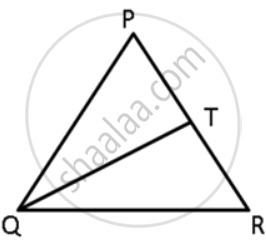Exercise 13.1 | Q 18.2

In the given figure, T is a point on the side PR of an equilateral triangle PQR. Show that RT < QTExercise 13.1 | Q 19

In ΔPQR is a triangle and S is any point in its interior. Prove that SQ + SR < PQ + PR.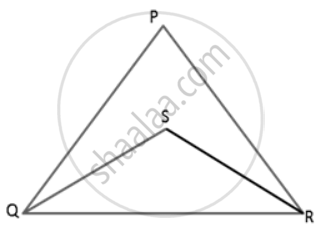Exercise 13.1 | Q 20

Prove that in an isosceles triangle any of its equal sides is greater than the straight line joining the vertex to any point on the base of the triangle.

Exercise 13.1 | Q 21

ΔABC in a isosceles triangle with AB = AC. D is a point on BC produced. ED intersects AB at E and AC at F. Prove that AF > AE.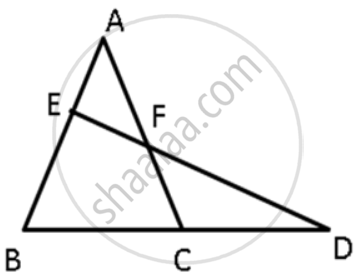Exercise 13.1 | Q 22

In ΔABC, AE is the bisector of ∠BAC. D is a point on AC such that AB = AD. Prove that BE = DE and ∠ABD > ∠C.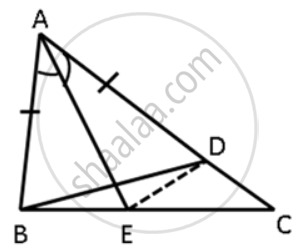Exercise 13.1 | Q 23

In ΔABC, D is a point in the interior of the triangle. Prove that DB + DC < AB + AC.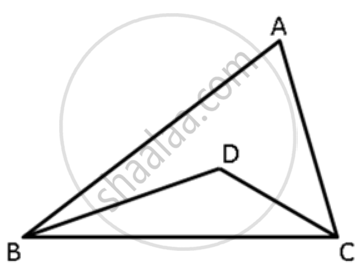## Chapter 13: Inequalities in Triangles

Exercise 13.1## Frank solutions for Class 9 Maths ICSE chapter 13 - Inequalities in Triangles

Frank solutions for Class 9 Maths ICSE chapter 13 (Inequalities in Triangles) include all questions with solution and detail explanation. This will clear students doubts about any question and improve application skills while preparing for board exams. The detailed, step-by-step solutions will help you understand the concepts better and clear your confusions, if any. Shaalaa.com has the CISCE Class 9 Maths ICSE solutions in a manner that help students grasp basic concepts better and faster.

Further, we at Shaalaa.com provide such solutions so that students can prepare for written exams. Frank textbook solutions can be a core help for self-study and acts as a perfect self-help guidance for students.

Concepts covered in Class 9 Maths ICSE chapter 13 Inequalities in Triangles are If two sides of a triangle are unequal, the greater side has the greater angle opposite to it., If Two Angles of a Triangle Are Unequal, the Greater Angle Has the Greater Side Opposite to It., Of All the Lines, that Can Be Drawn to a Given Straight Line from a Given Point Outside It, the Perpendicular is the Shortest., Inequalities in a Triangle.

Using Frank Class 9 solutions Inequalities in Triangles exercise by students are an easy way to prepare for the exams, as they involve solutions arranged chapter-wise also page wise. The questions involved in Frank Solutions are important questions that can be asked in the final exam. Maximum students of CISCE Class 9 prefer Frank Textbook Solutions to score more in exam.

Get the free view of chapter 13 Inequalities in Triangles Class 9 extra questions for Class 9 Maths ICSE and can use Shaalaa.com to keep it handy for your exam preparation